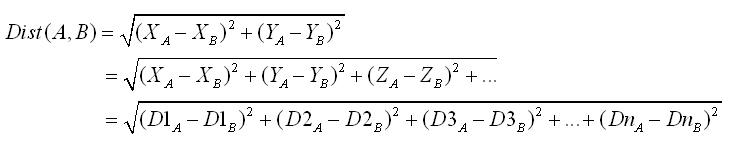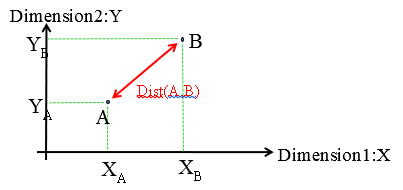﻿ 2. Introduction to the PCA techniques > 2.2. Distance definition > 2.2.3. All the type of distances available inside StarDust

# 2.2.3. All the type of distances available inside StarDust

A complete « segmentation model » is composed of:

oA list of  « centers  » of each segment.

oA definition of the « distance » used to compute the distance to each center.

Let’s have a closer look at the distances available inside StarDust.

With StarDust, you can mix several types of distances:

The Standard Euclidean Distance

The Pearson Distance (also named Cosine distance)

Each distance can be used inside different kinds of space:

The Original Space of the variable

The Quantile Space

The Space defined by the first axises of the PCA.

The pearson (or Cosine) distance is very useful when using Stardust to do text mining. When doing “textmining”, each row of a dataset represents a text-document.  The pearson (or Cosine) distance is commonly used to define distances between text-documents.

As a reminder, the Euclidean distance between the point A and B is expressed this way:It is simply the length of the red line here: